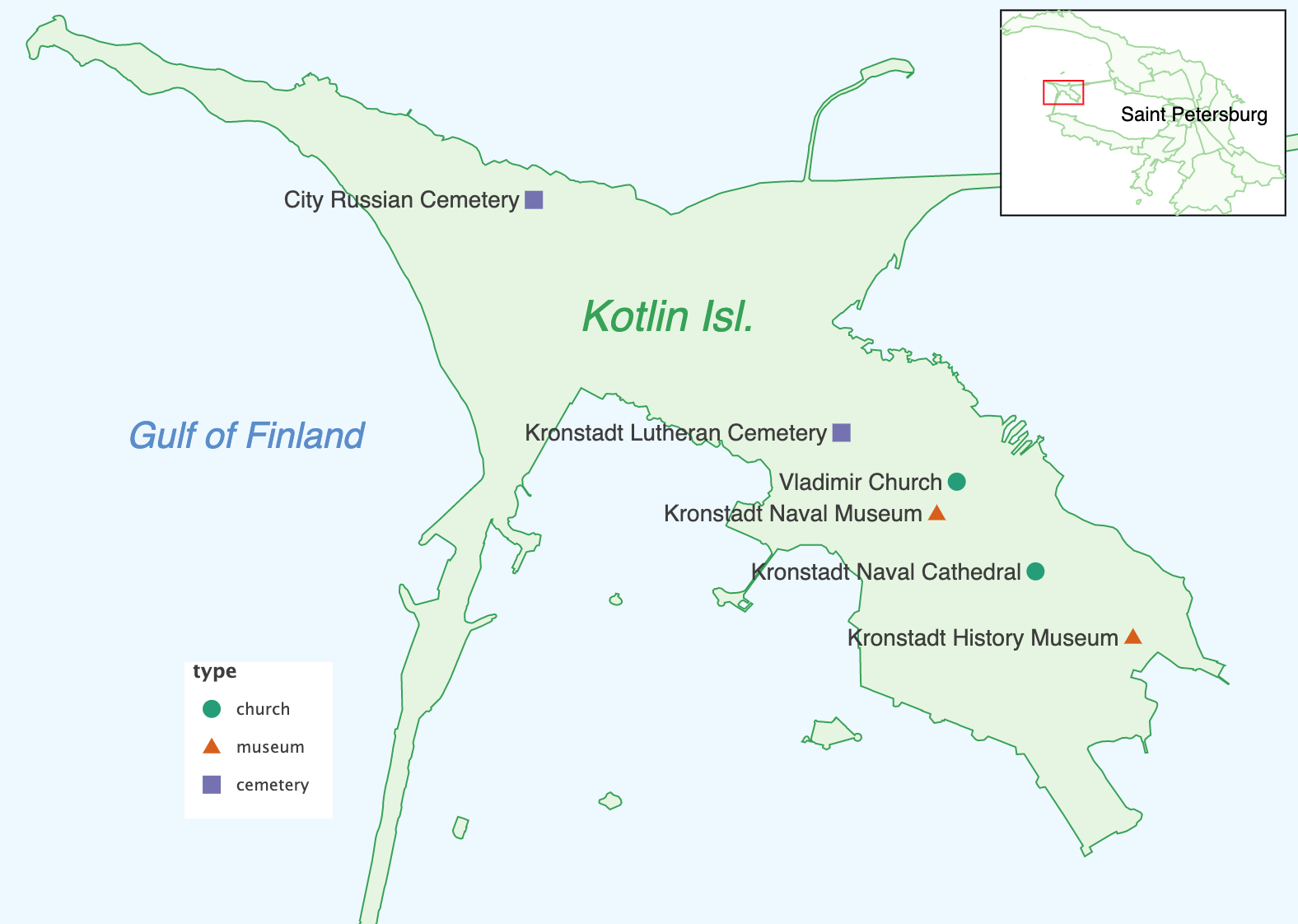# GeoPandas Support (GeoPandas and Shapely)¶

GeoPandas GeoDataFrame is a tabular data structure that contains a set of shapes (geometry) per each observation.

GeoDataFrame extends pandas DataFrame and as such, aside from the geometry, can contain other data.

GeoPandas supports the following three basic classes of geometric objects (shapes):

• Points / Multi-Points

• Lines / Multi-Lines

• Polygons / Multi-Polygons

All GeoPandas shapes are “understood” by Lets-Plot and can be plotted using various geometry layers, depending on the type of the shape.

Use:

## Plot Preliminaries¶

```import pandas as pd

from lets_plot.geo_data import *
from lets_plot import *
LetsPlot.setup_html()

df = pd.DataFrame({
'state': ['IL', 'IN', 'MI', 'OH', 'WI'],
'pop_2021': [12_569_321, 6_805_663, 9_992_427, 11_714_618, 5_852_490],
})
gdf = geocode_states(names=df.state).scope('US').get_boundaries()[['state', 'geometry']]
```
```The geodata is provided by © OpenStreetMap contributors and is made available here under the Open Database License (ODbL).
```

## Use Cases¶

Depending on the situation, for spatial data we use either parameter `data` or `map` or both.

### Use either `data` or `map` Parameter¶

Suppose we have the following data:

```gdf
```
state geometry
0 IL MULTIPOLYGON (((-89.20898 37.02010, -89.12109 ...
1 IN MULTIPOLYGON (((-87.62695 37.92687, -87.62695 ...
2 MI MULTIPOLYGON (((-88.41797 46.01222, -88.50586 ...
3 OH MULTIPOLYGON (((-82.17773 38.75408, -82.17773 ...
4 WI MULTIPOLYGON (((-92.81250 45.02695, -92.81250 ...
If you want to draw only shapes, then it makes no difference which parameter is used:
```ggplot() + geom_map(data=gdf)
```
```ggplot() + geom_map(map=gdf)
```

### Use `data` Parameter¶

If you want to use aesthetics, the `data` parameter is the only choice:

```ggplot() + geom_map(aes(fill='state'), color='white', data=gdf)
```

### Use Both: `data` and `map` Parameters¶

Suppose you also have a dataframe with population data:

```df
```
state pop_2021
0 IL 12569321
1 IN 6805663
2 MI 9992427
3 OH 11714618
4 WI 5852490
In this situation, in order to link aesthetics to the population numbers you will use:
• `data` parameter for the “population” dataframe,

• `map` parameter for the state boundaries `GeoDataframe`.

The 3rd parameter, `map_join`, will help to combine population values and state boundaries on the same chart:

```ggplot() + geom_map(aes(fill='pop_2021'), color='white', data=df, map=gdf, map_join='state')
```

## `use_crs` Parameter¶

Specify EPSG code of coordinate reference system (CRS). All coordinates in `GeoDataFrame` will be projected to this CRS.

```ggplot() + geom_map(map=gdf, use_crs="EPSG:32616")
```
Value “provided” tells Lets-Plot that the input `GeoDataframes` already contains coordinates in the desired CRS and should not be reprojected any further.
```gdf_utm = gdf.to_crs("EPSG:32616")
ggplot() + geom_map(map=gdf_utm, use_crs="provided")
```

## Interactive Maps¶

Apart from the `use_crs` parameter, `GeoDataFrames` are fully supported on Lets-Plot interactive maps.

When used in combination with `geom_livemap()`, the value of the `use_crs` parameter is ignored and all coordinates are reprojected using the Mercator projection.

```ggplot() + geom_livemap() + geom_map(aes(fill='state'), data=gdf, alpha=.5)
```

## Examples¶